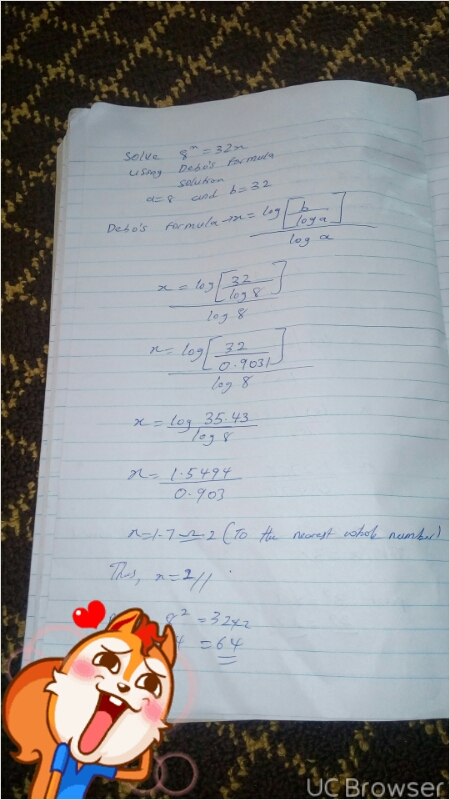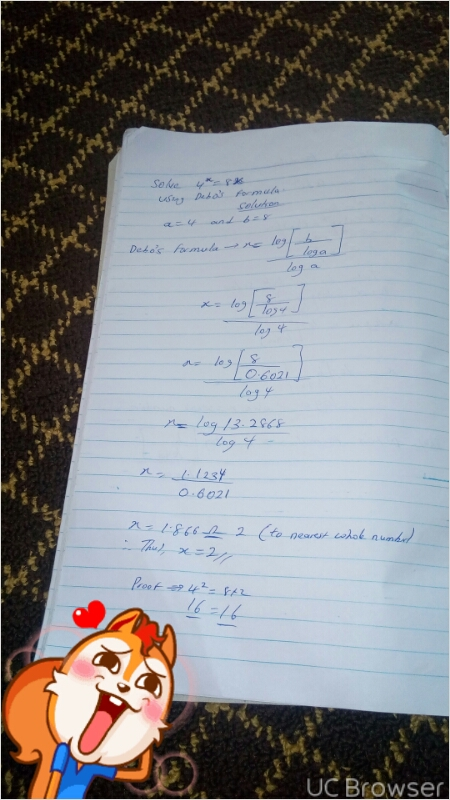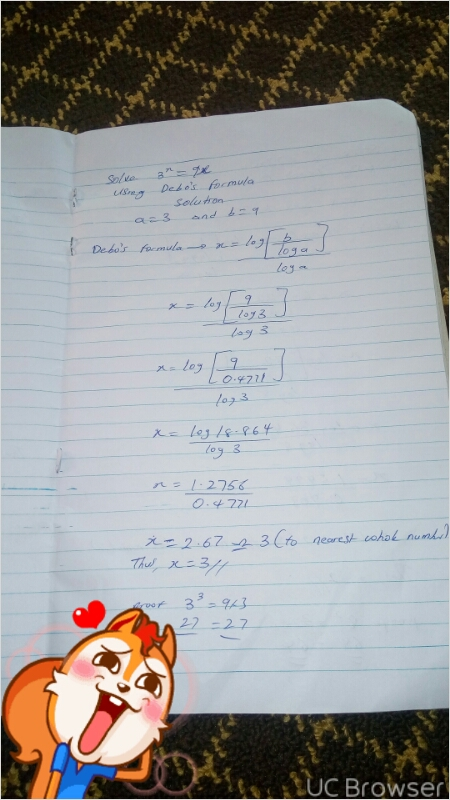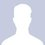# A 19 year old boy from Nigeria discovered his own formula in mathematics.

A 19 year old boy named Ayanlaja Ramoni Adebola from Nigeria has just discovered is own mathematical formula for solving mathematical problem of a^x=bx. A question was asked by his friend to him which brought his research to find the solution to the mathematical problem. He was asked to find the value of x in the equation 4^x=8x.He had to make research on such questions hoping they exist. But he discovered that most people know that the answer was 2 but what is the solution(workings) to the problem? Some made use of graph method, some made use of Eisten Raph method but he noticed most people had to limit the value of 2 because they already knew the answer was 2.

He made his research on such question and concluded there must be workings leading to the solution of the problem.

By his handwork he proposed his own formula which he named "Debo's formula". Which states that for the equation a^x=bx, then x={log{b/loga)} /loga. (where x is approximated to a whole number).

Chek out the solution of 4^x=8x using Debo's formula.
4^x=8x
Solution
a=4 and b=8
Debo's formula ==>x=log{b/loga} divided by log a
X=log{8/log4} /log4
X=log{8/0.6021} /log4
X=log 13.2868/log4
X=1.1234/0.6021
X=1.86=2(Approximate to the nearest whole number)
Thus, using Debo's formula x=2. Proof that x=2 from Debo's formula
4^x=8x
4^2=82
16=16
His friends argued that his formula can only be used to solve 4^x=8x...Another question of that type was created.
Solve 3^x=9x
Using Debo's formula
a=3 and b=9
x=log{b/loga} divided by loga x=log{9/log3) divided by log3
x=log(9/0.4771) /log3
x=log18. 864/log3
x=1.2756/0.4771
x=2.67=3(approximate to nearest whole number)
X=3 using Debo's formula.
Proof ==>
3^x=9x
3^3=9
3 27=27
Using Debo's formula
Another problem was created
8^x=32x
Using Debo's formula
a=8 and b=32
Debo's formula ==>x=log{b/loga} divided by loga
x=log{32/log8} /log8
x=log {32/0.9031}/log 8 X=log 35.43/log8
X=1.5494/0.903
X=1.7=2(Approximate to the nearest whole number)
Using Debo's formula x=2 Proof that x=2
8^x=32x
8^2=32 * 2
64=64
The conditions in using Debo's formula are both the LHS and RHS numeric value must be both even or both odd. Also the numeric value at exponential part (a^x) must be lesser and be divisible by the numeric value of the side with identity x. Thus in a^x=bx, a<b and a divisible by b to give a whole number. Lastly they must not be equal. So Debo later concluded that if the problem was in form of a^x=ax or b^x=bx or c^x=cx.. He concluded that x=1 must be among the solution to such problem.
Thus, 4^x=4x
Debo concluded that x=1 using his idea.
He later said Debo's formula can't be used to solve problems in form of a^x=ax cos the numeric digit of both Left hand side and Right hand side are equal because "a is in the left hand side and a is also on the right hand side" he concluded that x=1.
But if the equation is in form of a^x=bx
You can see that the numeric value of the Left hand side (a) is different from the numeric value of the right hand side =(b).
He concluded that Debo's formula is applicable to such equation.
For comments or observation or conversation concerning Debo's formula add him on Facebook with Ayanlaja Adebola Debo.5 years ago

This discussion board is a place to discuss our Daily Challenges and the math and science related to those challenges. Explanations are more than just a solution — they should explain the steps and thinking strategies that you used to obtain the solution. Comments should further the discussion of math and science.

When posting on Brilliant:

• Use the emojis to react to an explanation, whether you're congratulating a job well done , or just really confused .
• Ask specific questions about the challenge or the steps in somebody's explanation. Well-posed questions can add a lot to the discussion, but posting "I don't understand!" doesn't help anyone.
• Try to contribute something new to the discussion, whether it is an extension, generalization or other idea related to the challenge.

MarkdownAppears as
*italics* or _italics_ italics
**bold** or __bold__ bold
- bulleted- list
• bulleted
• list
1. numbered2. list
1. numbered
2. list
Note: you must add a full line of space before and after lists for them to show up correctly
paragraph 1paragraph 2

paragraph 1

paragraph 2

[example link](https://brilliant.org)example link
> This is a quote
This is a quote
    # I indented these lines
# 4 spaces, and now they show
# up as a code block.

print "hello world"
# I indented these lines
# 4 spaces, and now they show
# up as a code block.

print "hello world"
MathAppears as
Remember to wrap math in $$ ... $$ or $ ... $ to ensure proper formatting.
2 \times 3 $2 \times 3$
2^{34} $2^{34}$
a_{i-1} $a_{i-1}$
\frac{2}{3} $\frac{2}{3}$
\sqrt{2} $\sqrt{2}$
\sum_{i=1}^3 $\sum_{i=1}^3$
\sin \theta $\sin \theta$
\boxed{123} $\boxed{123}$

Sort by:

Awesome brother

- 5 years ago

Good stuff home boy . Keep up

- 5 years ago